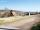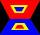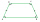Area of ditch

How great content area will have a section of trapezoidal ditch with a width of 1.6 meters above and below 0.57 meters? The depth of the ditch is 2.08 meters.

Result

x =  2.257 m2

Solution:Leave us a comment of example and its solution (i.e. if it is still somewhat unclear...):Be the first to comment!Next similar examples:

1. Trapezium basesFind the trapezium height if a = 8 cm and c = 4 cm if its content 21 square centimeters.
2. AnnulusThe radius of the larger circle is 8cm, the radius of smaller is 5cm. Calculate the contents of the annulus.
3. CableCable consists of 8 strands, each strand consists of 12 wires with diameter d = 0.5 mm. Calculate the cross-section of the cable.
4. Parcelparcel has a rectangular shape of a trapezoid with bases 12 m and 10 m and a height 8 m. On parcel was built object with a footprint an isosceles triangle shape with side 4 m and height three-quarters of a meter. What is the area of unbuild parcel?
5. 22/7 circleCalculate approximately area of a circle with radius 20 cm. When calculating π use 22/7.
6. Trapezium 2Trapezium has an area of 24 square cms. How many different trapeziums can be formed ?
7. TrapeziumDetermine the height of the trapezium ABCD, which has area 77.5 cm2 and base lengths 16 cm and 15 cm.
8. A trapezoidA trapezoid 75 ft wide on top 85 ft on the bottom, the height is 120 ft. What is its area in the square yds?
9. Trapezium zoomHow many times increase area of trapezoid if all sides and altitude increase 5 times?
10. AcreagePlot has a diamond shape, its side is 25.6 m long and the distance of the opposite sides is 22.2 meters. Calculate its acreage.
11. IronIron ore contains 57% iron. How much ore is needed to produce 20 tons of iron?
12. 4-gonIt is true that a 4-gon whose two sides are parallel and the other two has equal length, is a parallelogram?
13. Rectangular trapezoidHow many inner right angles has a rectangular trapezoid?
14. Isosceles trapezoid v3In an isosceles trapezoid ABCD is the size of the angle β = 81° Determine size of angles α, γ and δ.
15. Obtuse angleWhich obtuse angle is creating clocks at 17:00?
16. AverageThe arithmetic mean of the two numbers is 71.7. One number is 5. Calculate the second number.
17. ClassIn 7.C clss are 10 girls and 20 boys. Yesterday was missing 20% of girls and 50% boys. What percentage of students missing?